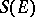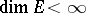# Totally-irreducible set

A setof linear operators (cf. Linear operator) on a locally convex topological vector spacethat is everywhere dense in the algebraof all weakly-continuous linear operators on(cf. Weak topology); in this contextis considered with the weak operator topology. The concept of a totally-irreducible set, initially introduced for Banach spaces, also proved useful in the theory of group representations, mainly for semi-simple Lie groups. Ifis a totally-irreducible set, it is also topologically irreducible, i.e. any closed subspace ofwhich is invariant with respect tocoincides with zero or with the entire space. Ifis a totally-irreducible set, its commutator subset inconsists of the operators that are multiples of the identity. The property of total irreducibility is equivalent to that of topological irreducibility in the following cases: 1); or 2)is a semi-group of unitary operators on a Hilbert space.